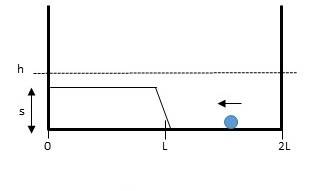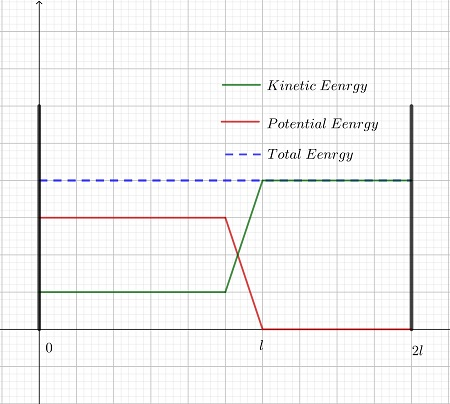A ball of mass m rolls back and forth without any loss of energy between two very high walls (at...

Question:

A ball of mass m rolls back and forth without any loss of energy between two very high walls (at x= 0 & x= 2L). There is a ramp centered at x= L that extends upward to a height s less than h. The ball has total energy {eq}E_{TOTAL} {/eq}= mgh (kinetic plus potential).

1) Is the total energy of the ball as it rolls from x= 2L to x= 0 increasing, decreasing, or staying the same? (Explain your answer)

2) Sketch below (all on one graph) the kinetic energy, gravitational potential energy, and total energy of the ball between x= 0 and x= 2L.Law of Conservation of Energy:

One of the most significant law in physics is the law of conservation of energy, which in simpler words, states that the energy can neither be created, nor be destroyed, but can only transform from one form to another.

For an isolated system, which can not exchange heat/energy from the surroundings, and is free from the influence of any external forces, the total energy of the system will not change with time. However, the different forms of the energies of the system may change as long as their sum is constant.

1)

The total energy of the ball would remain constant as there are no external forces in the region other than the force of gravity, for which the potential energy has already been considered. This is in accordance with the Law of Conservation of energy.

2)

The gravitational potential energy of an object with respect to the ground is:

{eq}U=mgx {/eq}

where {eq}m {/eq} is the mass of the object, {eq}g {/eq} is the acceleration due to gravity, and {eq}x {/eq} is the height of the object from the ground.

The kinetic energy at any point is simply equal to the difference between the total energy of the object and its gravitational potential energy.

{eq}K=E_{TOTAL}-U=mgh-mgx=mg\left (h-x \right ) {/eq}

The value of the three against the position of the ball, have been plotted below: